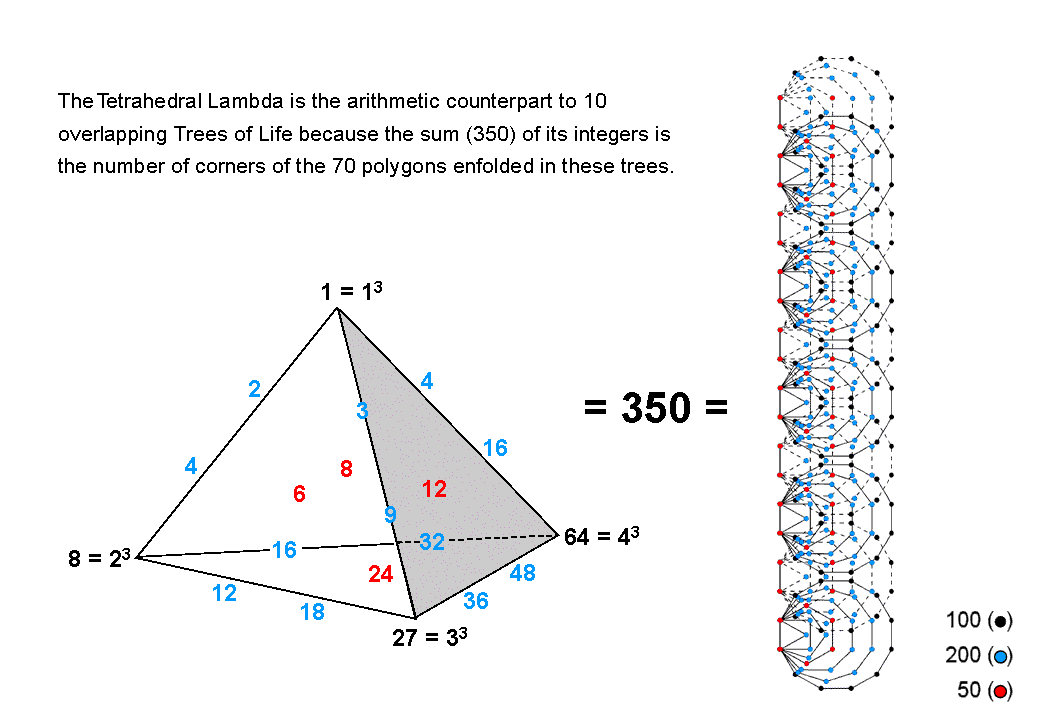<< Previous    1...   2  3    5  6  ...80    Next >>

#4 Correspondence between the Tetrahedral Lambda and the inner form of 10 overlapping Trees of LifeThe sum of the 20 integers in the Tetrahedral Lambda is 350. This is the number of corners that are intrinsic to the 70 polygons enfolded in 10 overlapping Trees of Life (the top corner of the hexagon enfolded in the 10th Tree of Life is the lowest corner of the hexagon enfolded in the 11th Tree and therefore is not intrinsic to polygons enfolded in the 10-tree). The sum of the black integers at the four vertices of the tetrahedron is 100. This is the number of black corners in the dodecagons outside their root edges. The sum of the red integers at the centres of the faces is 50. This is the number of red corners of the hexagons. The sum of the 12 remaining integers is 200. This is the number of blue corners outside the root edges of the triangles, squares, pentagons, octagons & decagons. Alternatively, there are:

1. 50 corners of triangles & squares and 200 corners of pentagons, hexagons, octagons & decagons;
2. 50 corners of pentagons and 200 corners of triangles, squares, hexagons, octagons & decagons.

In all three cases, the division of 50 into the pair of numbers 20 & 30 that is displayed by the Tetrahedral Lambda has its geometrical counterpart in the 20 endpoints of the 10 root edges and the 30 corners of polygons outside them. Which is the correct correspondence is an issue that need not detain us here. The crucial point is that the equivalence of the Tetrahedral Lambda and the inner form of 10 overlapping Trees of Life (a more differentiated representation of the 10 Sephiroth of a single Tree) is conclusive proof that the former is an object that has the same archetypal status as the Tree of Life. This is because the Tetrahedral Lambda is, in fact, the arithmetic counterpart of the inner form of these 10 Trees of Life.

 << Previous    1...   2  3    5  6  ...80    Next >>

Home